QUESTIONSHEET 1

(a) 3 A / 2                                                                                                                                                     1                             1

=  1.5 A                                                                                                                                                   1                             1

(b) 6 V                                                                                                                                                          1                             1

(c) resistance = V / I                                                                                                                                     1                           1

=  6 / 1.5                                                                                                                                                  1                             1

=  4 W                                                                                                                                                     1                                  1

TOTAL  / 6

QUESTIONSHEET 2

(a) 5 W  +  10 W                                                                                                                                                1                             1

= 15 W                                                                                                                                                     1                                  1

(b) I = V / R                                                                                                                                                  1                             1

= 3 / 15                                                                                                                                                    1                             1

= 0.2 A                                                                                                                                                    1                             1

Units are essential in calculations.  Sometimes examination questions give a blank space for the answer followed by the unit.  Sometimes no unit is given and you are expected to supply it.  It is a common mistake to leave the units out altogether – don’t get into this bad habit.

TOTAL  / 5

QUESTIONSHEET 3

 6v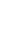(a)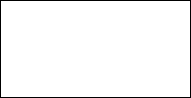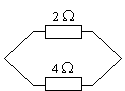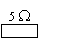symbols correct                                                                                                                                   1                           1

2 W and 4 W resistors in parallel                                                                                                           1                    1

5 W resistor in series                                                                                                                            1                           1

(b) resistance of parallel resistors given by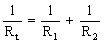1                         1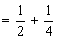1                             1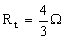1                             1

so total resistance =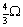+ 5 =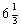W                                                                                                         1                    1

(c)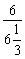1                             1

= 0.95 A                                                                                                                                                  1                             1

For parallel resistors, it is sometimes easier to use the expression  Product / Sum for the total resistance.

i.e. here we get 2 × 4 / (2 + 4)  = 8 / 6  =  4/3 W

TOTAL  / 9

QUESTIONSHEET 4

(a) (i)    Power of lamp is 6 W                                                                                                                       1                             1

when used on 12 V                                                                                                                           1                             1

(ii)    6 W  =  6 J/s                                                                                                                                     1                                  1

5 minutes = 300 s                                                                                                                              1                             1

energy transferred  =  300 ´ 6                                                                                                           1                           1

= 1800 J                                                                                                                                           1                                  1

(b) (i)    I  =  P / V   =  6 / 12                                                                                                                         1                             1

=  0.5 A                                                                                                                                           1                                  1

(ii)    R  =  V / I   =  12 / 0.5                                                                                                                      1                             1

=  24 W                                                                                                                                                1                                  1

(c) No difference                                                                                                                                           1                                  1

24 V shared between 2 lamps / pd across each lamp is 12 V                                                                       1

Power is the rate of transferring electrical energy into other forms – in this case, heat and light.  A 6 W lamp transfers 6 Joules of electrical energy into heat & light each second, since 1 W = 1 J/s.

The formula  P = I / V is one you need to know.

TOTAL  / 12

QUESTIONSHEET 5

(a) (i)    0.6 ´ 10/20                                                                                                                                       1                             1

= 0.3 A                                                                                                                                                 1                             1

(ii)    0.6 + 0.3                                                                                                                                           1                             1

=  0.9 A                                                                                                                                           1                             1

(b)        V = I R                                                                                                                                                1                             1

0.6 ´ 10 or 0.3 ´ 20                                                                                                                           1                           1

=  6 V                                                                                                                                              1                                  1

Current is inversely proportional to the resistance as long as the pd is constant. So half the current flows through the resistor that is twice as big.

TOTAL  / 7

QUESTIONSHEET 6

(a)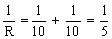1                           1

R = 5 W                                                                                                                                                       1                             1

(b) 10 + 5                                                                                                                                                          1                             1

= 15 W                                                                                                                                                     1                             1

(c) current through single resistor  = V / R = 6 / 15                                                                                          1

=  0.4 A                                                                                                                                                   1                             1

current through parallel resistors = 0.2 A                                                                                                    1

In many questions, the value of the parallel resistors is the same.  In this case, the total resistance is half the value of one.

TOTAL  / 7

QUESTIONSHEET 7

(a) (i)    2 + 5 + 10                                                                                                                                         1                                  1

= 17 W                                                                                                                                             1                                       1

(ii)    1/R = 1/2 + 1/5 + 1/10                                                                                                                       1                             1

= 8 / 10 W                                                                                                                                        1                                  1

R = 10 / 8 = 1.25 W                                                                                                                           1                             1

(b) Current larger in (ii)                                                                                                                                  1                             1

current inversely proportional to resistance                                                                                                 1                    1

smaller resistance means larger current                                                                                                     1                    1

TOTAL  / 8

QUESTIONSHEET 8

(a)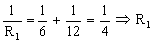= 4W                                                                                                                 1                             1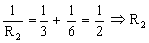= 2                                                                                                                    1

Total resistance = 2 + 4 = 6W                                                                                                                    1                           1                                                                                                                                                                               1

(b) I = V / R                                                                                                                                                  1                                  1

=  12 / 6                                                                                                                                                   1                                  1

= 2 A                                                                                                                                                       1                                       1

(c) R = 4 + 3                                                                                                                                                     1                                  1

=  7                                                                                                                                                         1                                  1

I  =  12 / 7  or 1.7 A                                                                                                                                 1                             1

(d) R = 3 + 6  =  9 W                                                                                                                                     1                             1

I = V / R  =  12 / 9                                                                                                                                   1                             1

=  1.33 A                                                                                                                                                     1                                  1

TOTAL  / 11

QUESTIONSHEET 9

(a) C                                                                                                                                                             1                             1

(b) (i)  a complete path                                                                                                                                   1                           1

for electricity to flow                                                                                                                            1                           1

when connected to a voltage supply                                                                                                      1                    1

(ii) A & D (both)                                                                                                                                      1                             1

No electricity flows in the last circuit, because the cells are the opposite way round from each other,

so they cancel out.

TOTAL  / 5

QUESTIONSHEET 10

(a) switch shown in either of the positions indicated                                                                                         1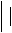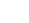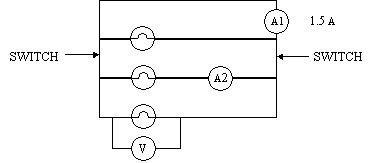(b) Parallel                                                                                                                                                    1                             1

(c) 1.5 / 3                                                                                                                                                          1                                  1

=  0.5 A                                                                                                                                                   1                                       1

(d) 12 V                                                                                                                                                            1                                                                                                                                                                              1

TOTAL  / 5

QUESTIONSHEET 11

(a) Resistance in circuit alters current                                                                                                             1                         1

ammeter resistance must be low so it doesn’t affect

current flow                                                                                                                                             1                             1

(b) (i)    In parallel across component                                                                                                              1                           1

(ii)    voltmeter must have high resistance                                                                                                   1                         1

little current flows through voltmeter                                                                                                  1                         1

most current flows through component                                                                                               1                    1

For two parallel resistors, 1/R  =  1/R1  +  1/R2.  So if one resistor (the voltmeter) R2 has a high resistance, 1/R2 is very small and the total resistance is almost the same as without the voltmeter.

TOTAL  / 6

QUESTIONSHEET 12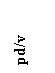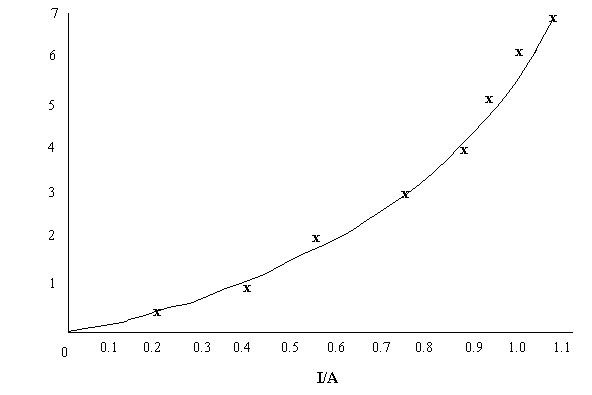(a)

axes labelled + units                                                                                                                   1                             1

correct plotting                                                                                                                           2                             2

smooth curve drawn                                                                                                                   1                             1

(b) (i)    no                                                                                                                                                    1                                  1

(ii)    the graph is not a straight line                                                                                                             1                           1

or V / A  ¹  R                                                                                                                                  1                                  1

(iii)   filament lamp                                                                                                                                    1                                  1

TOTAL  / 8

QUESTIONSHEET 13

(a) Voltage is proportional to current                                                                                                               1

for a metal conductor at constant temperature                                                                                            1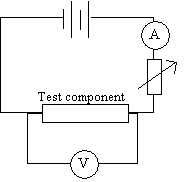(b)

circuit symbols correct                                                                                                                         1

ammeter in series                                                                                                                                1

voltmeter in parallel                                                                                                                              1

variable resistor in series                                                                                                                      1

TOTAL  / 6

QUESTIONSHEET 14

(a) A  =  metal wire                                                                                                                                       1                             1

B  =  diode                                                                                                                                               1                                  1

C  =  lamp filament                                                                                                                                   1                             1

(b) A                                                                                                                                                            1                                       1

(c) C                                                                                                                                                             1

An ohmic conductor obeys Ohm’s law, so a graph of I against V must be a straight line through the origin.

The gradient of graph C decreases so the resistance must be increasing.

TOTAL  / 5

QUESTIONSHEET 15

(a) (i)

 MACHINE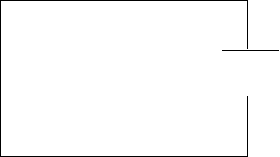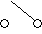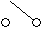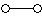two switches                                                                                                                              1                                  1

in series                                                                                                                                     1                                  1

with machine                                                                                                                             1                                  1

(ii)    each switch must be able to turn off machine                                                                                      1                    1

(b) switches not in series                                                                                                                                1                             1

each must be able to switch light on or off                                                                                                  1                    1

needs two parallel parts of circuit                                                                                                              1                         1

TOTAL  / 7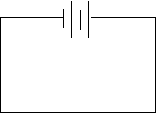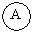QUESTIONSHEET 16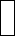(a) Set up Ohm’s law circuit                                                                                                                           1                         1

 Test component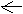measure pd across wire and current through it                                                                                            1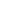measure length of wire                                                                                                                             1                           1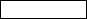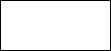change length of wire and repeat experiment                                                                                              1                             1                                                                                                                                                                         1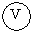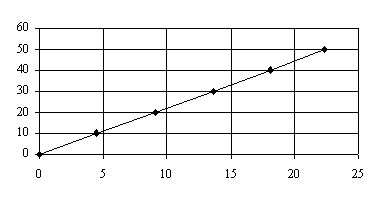(b) (i)

 Length (cm)

 Resistance (W)

(ii)    as length increases                                                                                                                            1

resistance increases

directly proportional                                                                                                                           1

(iii)   as length varies, resistance varies                                                                                                       1                         1

current varies                                                                                                                                   1                           1

and light dims or brightens                                                                                                                 1                           1

The first two or three marks of this question could be obtained by drawing a labelled diagram.

TOTAL  / 12

QUESTIONSHEET 17

(a) A  =  battery                                                                                                                                                1                             1

B  =  lamp/light bulb                                                                                                                                  1                           1

C  =  switch                                                                                                                                             1                             1

D  =  variable resistor                                                                                                                               1                           1

(b) varies current in a circuit / acts as dimmer switch                                                                                       1

You must be able to recognise circuit components.  Look at the syllabus or ask your teacher which ones you must know.  Note that a battery is made up of two or more cells joined in series

TOTAL  / 5

QUESTIONSHEET 18

(a) (i)    1.5 V                                                                                                                                               1                                       1

(ii)    0 V                                                                                                                                                  1                                  1

(iii)   3.0 V                                                                                                                                               1                                       1

(iv)   1.5 V                                                                                                                                               1                                       1

(v)   1.5 V                                                                                                                                               1                                       1

(b) car bulb needs 12 v                                                                                                                                   1                             1

(c) increases                                                                                                                                                 1                                  1

TOTAL  / 7

QUESTIONSHEET 19

(a) silver too expensive                                                                                                                                  1                              1

(b) copper is too heavy                                                                                                                                   1                             1

(c) rubber and PVC                                                                                                                                       2                           1

(d) water will conduct electricity                                                                                                                     1                           1

would get shock with wet hands                                                                                                                1                         1

TOTAL  / 7

QUESTIONSHEET 20

(a) One connected after another in line                                                                                                           2                    1

(b) 240 / 20                                                                                                                                                   1                                  1

=  12 V                                                                                                                                                    1                                       1

(c) bulb from 40-bulb set uses 6 v                                                                                                                   1                         1

higher voltage would cause it to blow                                                                                                         1                         1

(d) (i)    fuse would blow repeatedly                                                                                                               1                           1

(ii)    fault would not blow fuse                                                                                                                   1                           1

could be dangerous to operator                                                                                                          1                         1

TOTAL  / 9